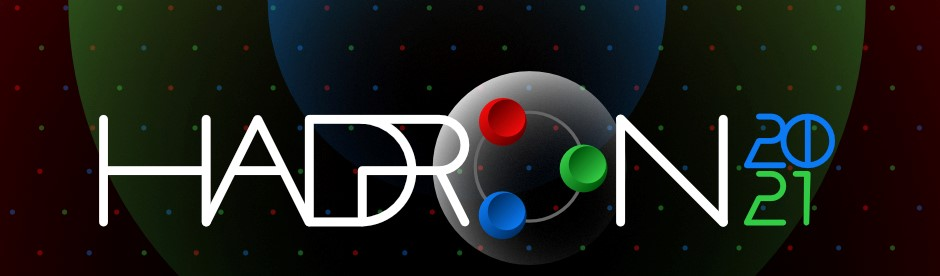#19th International Conference on Hadron Spectroscopy and Structure in memoriam Simon Eidelman

26-31 July 2021
Mexico City
Mexico/General timezone
HADRON 2021 is over. Thanks for making it a success!
Home > Timetable > Session details > Contribution details

# Contribution Parallel

Mexico City -
Hadron Decays, Production and Interactions

# Ratios of Partial Wave Amplitudes in the Decays of $J=1$ and $J=2$ Mesons.

## Speakers

• Dr. Vanamali SHASTRY

## Abstract

We study the two body decay of mesons using the helicity formalism. We find that to explain the ratio of partials wave amplitudes (PWAs) of the decay, the Lagrangian must include derivative interactions in addition to contact interactions. We estimate the coupling constants for the $1^{++}\to1^{--}0^-$, $1^{+-}\to1^{--}0^-$, $2^{-+}\to2^{++}0^-$, and $2^{-+}\to1^{--}0^-$ decays by fitting the ratio of the PWAs and the decay widths to the available data and predict the ratios to some new decays.